# MA.4.FR.2.4Export Print
Extend previous understanding of multiplication to explore the multiplication of a fraction by a whole number or a whole number by a fraction.

### Examples

Example: Shanice thinks about finding the product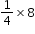by imagining having 8 pizzas that she wants to split equally with three of her friends. She and each of her friends will get 2 pizzas since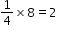.

Example: Lacey thinks about finding the product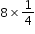by imagining having 8 pizza boxes each with one-quarter slice of a pizza left. If she put them all together, she would have a total of 2 whole pizzas since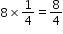which is equivalent to 2.

### Clarifications

Clarification 1: Instruction includes the use of visual models or number lines and the connection to the commutative property of multiplication. Refer to Properties of Operation, Equality and Inequality (Appendix D).

Clarification 2: Within this benchmark, the expectation is not to simplify or use lowest terms.

Clarification 3: Fractions multiplied by a whole number are limited to less than 1. All denominators are limited to 2, 3, 4, 5, 6, 8, 10, 12, 16, 100.

General Information
Subject Area: Mathematics (B.E.S.T.)
Strand: Fractions
Status: State Board Approved

## Benchmark Instructional Guide

• Equation
• Expression

### Vertical Alignment

Previous Benchmarks

Next Benchmarks

### Purpose and Instructional Strategies

The purpose of this benchmark is to connect previous understandings of whole-number multiplication concepts (MA.3.NSO.2.2) and apply them to the multiplication of fractions. This work builds a foundation for multiplying fractions with procedural reliability in grade 5 (MA.5.FR.2.2).
• Contexts involving multiplying whole numbers and fractions lend themselves to modeling and examining patterns.
• This benchmark builds on students’ work of adding fractions with like denominators (MA.4.FR.2.2) and extending that work into the relationship between addition and multiplication.
• Students should use fraction models and drawings to show their understanding. Fraction models may include area models, number lines, set models, or equations.
• During instruction, teachers should relate “ total group size” language that was used to introduce whole number multiplication- possibly changing from “ total group size” to “ total size” or “ total amount” (see Appendix A). Using such language, the expression 5 ×$\frac{\text{3}}{\text{4}}$ can be described as the total size or amount of 5 objects, each of which has size or amount of $\frac{\text{3}}{\text{4}}$
• For example, the weight of 5 slabs of chocolate that each weigh $\frac{\text{3}}{\text{4}}$ of a pound is 5 × $\frac{\text{3}}{\text{4}}$ pounds. Students need to understand that when multiplying a whole number by a fraction, the most important idea is that the whole number describes the number of objects and the fraction describes the size of each object.
• Instruction should include representing a whole number times a fraction as repeated addition: 5×$\frac{\text{3}}{\text{4}}$ = $\frac{\text{3}}{\text{4}}$ + $\frac{\text{3}}{\text{4}}$ + $\frac{\text{3}}{\text{4}}$ + $\frac{\text{3}}{\text{4}}$ + $\frac{\text{3}}{\text{4}}$
• When multiplying a fraction by a whole number, teachers can use language like “portion of” to convey that the fraction represents the “portion of” the whole number.
• For example, the $\frac{\text{3}}{\text{4}}$ portion of a 5 mile run is $\frac{\text{3}}{\text{4}}$ × 5 miles.
• Instruction includes fractions equal to and greater than one.
• Exploring patterns of what happens to the numerator when a whole number is multiplied by a fraction will help students make sense of multiplying fractions by fractions in Grade 5. When multiplying whole numbers by mixed numbers, students can use the distributive property or write the mixed number as a fraction greater than one. During instruction, students should compare both strategies. Using the distributive property to multiply a whole number by a mixed number could look like this.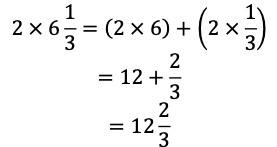### Common Misconceptions or Errors

• Students may multiply both the numerator and the denominator by the whole number. It is important to provide students with visual models, manipulatives or context to model the computation.
• Students may be confused by the fact that a fraction times a whole number often represents something quite different than a whole number times a fraction, even though the commutative property says the order does not affect the value.
• Without a conceptual understanding of how fraction multiplication is modeled, students can be confused regarding why the denominator remains the same when multiplying a whole number by a fraction. During instruction, teachers should relate fraction multiplication to repeated addition to explain why only the numerator changes.

### Strategies to Support Tiered Instruction

• Instruction includes connecting repeated addition and models to understand the meaning of the factors in a multiplication equation.
• For example, 8 × $\frac{\text{1}}{\text{4}}$ can be shown using repeated addition with 8 groups of $\frac{\text{1}}{\text{4}}$
• $\frac{\text{1}}{\text{4}}$ + $\frac{\text{1}}{\text{4}}$ + $\frac{\text{1}}{\text{4}}$ + $\frac{\text{1}}{\text{4}}$ + $\frac{\text{1}}{\text{4}}$ + $\frac{\text{1}}{\text{4}}$ + $\frac{\text{1}}{\text{4}}$ + $\frac{\text{1}}{\text{4}}$ = $\frac{\text{8}}{\text{4}}$ or 2 wholes
• For example, $\frac{\text{1}}{\text{4}}$ × 8 can be shown as $\frac{\text{1}}{\text{4}}$ of 8 using a number line.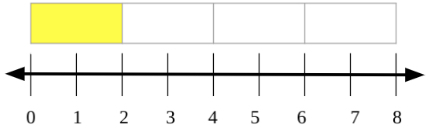• Instruction includes real-world situations aligned with the content. The teacher provides a multiplication expression with real-world context and items to represent the situation to make connections.
• For example, the teacher provides the student with the following situation: “The theater class has to make costumes that require $\frac{\text{1}}{\text{4}}$ foot of ribbon. They need to make 8 costumes. How many feet of ribbon will they need total?” The teacher provides ribbon already cut into $\frac{\text{1}}{\text{4}}$ foot pieces and has students model the problem. Students then write out the repeated addition problem to match $\frac{\text{1}}{\text{4}}$ + $\frac{\text{1}}{\text{4}}$ + $\frac{\text{1}}{\text{4}}$ + $\frac{\text{1}}{\text{4}}$ + $\frac{\text{1}}{\text{4}}$ + $\frac{\text{1}}{\text{4}}$ + $\frac{\text{1}}{\text{4}}$+ $\frac{\text{1}}{\text{4}}$ = $\frac{\text{8}}{\text{4}}$ or 2 feet.
• For example, the teacher provides the student with the following situation: “The theater class has 8 feet of ribbon. They need to make 4 costumes and they want to use up all of the ribbon. How many feet of ribbon does each costume use?” The teacher will provide the student with a piece of ribbon 8 feet long and have them mark it off into 4 equal sections and find the length of 1 section (2 feet).
• Teacher provides models that represent multiplication expressions to represent the Commutative Property of Multiplication and has students explain the difference in meaning.
• For example, the teacher provides the student with the following models.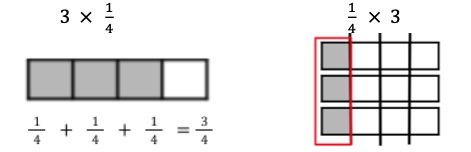• The teacher provides models and asks students which expression it models.
• For example, the teacher provides students with the following model and asks if it represents $\frac{\text{1}}{\text{6}}$ x 4 or 4 x $\frac{\text{1}}{\text{6}}$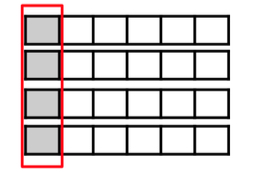How many $\frac{\text{2}}{\text{5}}$ are in $\frac{\text{12}}{\text{5}}$ ? Use a visual model to explain your reasoning and show the relationship to the multiplication of a whole number by a fraction.

### Instructional Items

Instructional Item 1

An expression is shown.
$\frac{\text{3}}{\text{4}}$ x 9

What is the product?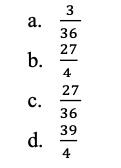Instructional Item 2

Choose all the ways to express the product of $\frac{\text{3}}{\text{4}}$ × 5.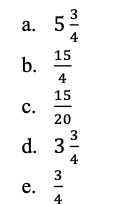*The strategies, tasks and items included in the B1G-M are examples and should not be considered comprehensive.

## Related Courses

This benchmark is part of these courses.
5012060: Mathematics - Grade Four (Specifically in versions: 2014 - 2015, 2015 - 2022, 2022 and beyond (current))
7712050: Access Mathematics Grade 4 (Specifically in versions: 2014 - 2015, 2015 - 2018, 2018 - 2022, 2022 and beyond (current))
5012065: Grade 4 Accelerated Mathematics (Specifically in versions: 2019 - 2022, 2022 and beyond (current))
5012015: Foundational Skills in Mathematics 3-5 (Specifically in versions: 2019 - 2022, 2022 and beyond (current))

## Related Access Points

Alternate version of this benchmark for students with significant cognitive disabilities.
MA.4.FR.2.AP.4: Explore the multiplication of a unit fraction by a whole number (e.g., 3 ×, 2 ×, 5 ×). Denominators limited to 2, 3, 4, 6, 8 or 10.

## Related Resources

Vetted resources educators can use to teach the concepts and skills in this benchmark.

## Educational Game

Ice Ice Maybe: An Operations Estimation Game:

This fun and interactive game helps practice estimation skills, using various operations of choice, including addition, subtraction, multiplication, division, using decimals, fractions, and percents.

Various levels of difficulty make this game appropriate for multiple age and ability levels.

Multiplication/Division: The multiplication and addition of whole numbers.

Percentages: Identify the percentage of a whole number.

Fractions: Multiply and divide a whole number by a fraction, as well as apply properties of operations.

Type: Educational Game

## Formative Assessments

How Much Sugar?:

Students are asked to multiply a fraction by a whole number to solve a word problem and to represent the product with a visual fraction model.

Type: Formative Assessment

Training for a Race:

Students are asked to multiply an improper fraction by a whole number to solve a word problem and use a visual model or equation to represent the problem.

Type: Formative Assessment

How Many One Fourths?:

Students are asked to multiply a fraction by a whole number and to represent the product with a visual fraction model.

Type: Formative Assessment

Multiplying Fractions by Whole Numbers:

Students are asked to consider an equation involving multiplication of a fraction by a whole number and create a visual fraction model. Additionally, the student is asked to interpret multiplying the number of parts by the whole number.

Type: Formative Assessment

Fractions and Multiples:

Students use a visual fraction model to explain how many one sixths are in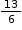and record their work with an equation.

Type: Formative Assessment

## Image/Photograph

Clipart ETC Fractions:

Illustrations that can be used for teaching and demonstrating fractions. Fractional representations are modeled in wedges of circles ("pieces of pie") and parts of polygons. There are also clipart images of numerical fractions, both proper and improper, from halves to twelfths. Fraction charts and fraction strips found in this collection can be used as manipulatives and are ready to print for classroom use.

Type: Image/Photograph

## Lesson Plans

Lessen the Litter:

Students will calculate the total amount of trash at different locations in the community to determine which location has the most trash and explore ways a community can work together to prevent future trash buildup in this integrated lesson plan.

Type: Lesson Plan

Volunteering with the Mayor Part 2:

Volunteering is vital to keeping any community safe, inviting, and running smoothly! In this lesson, students will work together to plan a volunteer project they would like to see happen in their community; as well as, create a budget for their project.

Type: Lesson Plan

Volunteering with the Mayor:

The mayor wants to build a new park in town! Volunteer your time and help the mayor design an expense report with a given budget for the new park in this lesson.

Type: Lesson Plan

Modeling Multiple Groups of Fractions:

In this inquiry lesson students will use a situational story to explore ways to find the total quantity of a fraction multiplied by a whole number using various models.

Type: Lesson Plan

Multiple Bake Sale Cookie Recipes with fractional ingredients:

In this lesson students will explore ways to find the product of mixed numbers multiplied by a whole number using a real-world situation.

Type: Lesson Plan

"What's the part? What's the whole?":

This lesson provides a conceptual approach to multiplying a fraction times a whole number and a whole number times a fraction.  Students are to use an understanding of the meaning of the denominator and numerator to figure out a strategy for finding the solution.

Type: Lesson Plan

Looking for Patterns in a Sequence of Fractions:

Students generate and describe a numerical pattern using the multiplication and subtraction of fractions.

Type: Lesson Plan

Exploring Fraction Multiplication:

Students will link multiplication of a whole number times a fraction with repeated addition and fraction circle manipulative.

Type: Lesson Plan

Modeling Multiplication with Fractions:

Students will relate multiplication strategies with fractions through problem solving situations. This lesson connects prior understanding of multiplication and equal groups to multiplication of fractions.

Type: Lesson Plan

Multiple Bake Sale Cookie Recipes with fractional ingredients PART 1:

In this lesson students are guided through the process of multiplying a whole number and a fraction in a real-world situation.  The lesson uses the number line to explain the process.

Type: Lesson Plan

Multiply Fractions and Whole Numbers with Models:

Students will multiply a whole number by a fraction through set models and problem solving.

Type: Lesson Plan

## Original Student Tutorial

Multiplying Fractions with Bake Sale Mania:

Find the total amounts of repeated fraction quantities by multiplying a fraction by a whole number using visual models that represent real-world problems and cookies in this interactive tutorial.

Type: Original Student Tutorial

## Perspectives Video: Teaching Idea

Exploring Fractions with Pattern Blocks:

Unlock an effective teaching strategy for using pattern blocks to explore fraction concepts in this Teacher Perspectives video for educators.

Type: Perspectives Video: Teaching Idea

Sugar in six cans of soda:

This task provides a familiar context allowing students to visualize multiplication of a fraction by a whole number. This task could form part of a very rich activity which includes studying soda can labels.

Connor and Makayla Discuss Multiplication:

The purpose of this task is to have students think about the meaning of multiplying a number by a fraction, and use this burgeoning understanding of fraction multiplication to make sense of the commutative property of multiplication in the case of fractions.

## Tutorial

Multiplying a Fraction by a Whole Number:

In this Khan Academy video visual fraction models are used to represent the multiplication of a whole number times a fraction.

Type: Tutorial

## MFAS Formative Assessments

Fractions and Multiples:

Students use a visual fraction model to explain how many one sixths are inand record their work with an equation.

How Many One Fourths?:

Students are asked to multiply a fraction by a whole number and to represent the product with a visual fraction model.

How Much Sugar?:

Students are asked to multiply a fraction by a whole number to solve a word problem and to represent the product with a visual fraction model.

Multiplying Fractions by Whole Numbers:

Students are asked to consider an equation involving multiplication of a fraction by a whole number and create a visual fraction model. Additionally, the student is asked to interpret multiplying the number of parts by the whole number.

Training for a Race:

Students are asked to multiply an improper fraction by a whole number to solve a word problem and use a visual model or equation to represent the problem.

## Original Student Tutorials Mathematics - Grades K-5

Multiplying Fractions with Bake Sale Mania:

Find the total amounts of repeated fraction quantities by multiplying a fraction by a whole number using visual models that represent real-world problems and cookies in this interactive tutorial.

## Student Resources

Vetted resources students can use to learn the concepts and skills in this benchmark.

## Original Student Tutorial

Multiplying Fractions with Bake Sale Mania:

Find the total amounts of repeated fraction quantities by multiplying a fraction by a whole number using visual models that represent real-world problems and cookies in this interactive tutorial.

Type: Original Student Tutorial

## Educational Game

Ice Ice Maybe: An Operations Estimation Game:

This fun and interactive game helps practice estimation skills, using various operations of choice, including addition, subtraction, multiplication, division, using decimals, fractions, and percents.

Various levels of difficulty make this game appropriate for multiple age and ability levels.

Multiplication/Division: The multiplication and addition of whole numbers.

Percentages: Identify the percentage of a whole number.

Fractions: Multiply and divide a whole number by a fraction, as well as apply properties of operations.

Type: Educational Game

Sugar in six cans of soda:

This task provides a familiar context allowing students to visualize multiplication of a fraction by a whole number. This task could form part of a very rich activity which includes studying soda can labels.

Connor and Makayla Discuss Multiplication:

The purpose of this task is to have students think about the meaning of multiplying a number by a fraction, and use this burgeoning understanding of fraction multiplication to make sense of the commutative property of multiplication in the case of fractions.

## Tutorial

Multiplying a Fraction by a Whole Number:

In this Khan Academy video visual fraction models are used to represent the multiplication of a whole number times a fraction.

Type: Tutorial

## Parent Resources

Vetted resources caregivers can use to help students learn the concepts and skills in this benchmark.

## Image/Photograph

Clipart ETC Fractions:

Illustrations that can be used for teaching and demonstrating fractions. Fractional representations are modeled in wedges of circles ("pieces of pie") and parts of polygons. There are also clipart images of numerical fractions, both proper and improper, from halves to twelfths. Fraction charts and fraction strips found in this collection can be used as manipulatives and are ready to print for classroom use.

Type: Image/Photograph